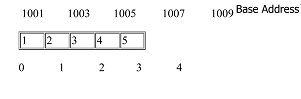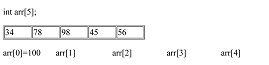# C++ language

Arrays IN C++
 Previous Home Next

An array is a collection of element of same data types.Arrays are stored linearly in memory. For example, if we have to store 7 values of any type, lets say, integer values we don't have to declare 5 variables with different identifiers but we can define an array of integer type and can store the values in array directly with a unique identifier. Thus, an array named arr created in memory having 5 values can be represented as :HereIndex no. is the position of array where data can be stored, i.e. 0,1,2,3,4.

Note: In array indexing stqart from 0 to (n-1) , where n is the number of bit in array.

### Address Space:

 Data Type Address Space Char, 1 Int, 2 Float, 4 Double, 8 Long, 10

Array memory size: This can be determined by the formula

Array memory size = Size * <No. of byte allocated to data type>
For Example
``` int a
Array memory size = 10 * 2
= 20
```

### Declaring Arrays:

Syntax
```< data _type >  < array _name > [ size ]  ;
eg: int a  ;
```
How to initialize an array:

While declaring the array for a local scope e.g. for a particular function its contents will not be initialized by default and will remain undetermined till we initialized it separately. In case of array of global scope the values are initialized but having the default values of 0's for each element.

Thus, for the array of both scopes we can initialize an array by enclosing the elements within the {} brackets. For example,

int arr = {1,2,3,4,5}; /* This array will be created as which one is shown in the above figure. */

The no. of elements declared within the {} must not exceeds the numbers of elements defined in the [], else it could some serious trouble to your program.

### Advantages of using an array:

1. Arrays are the most convenient way of storing the fixed amount of data that can be accessed in an unpredictable fashion.
2. Arrays are also one of the most compact data structures as in for storing the 50 elements in an array of integer type it will take the memory equivalent to the amount of memory required to store 50 elements in addition to that a few overhead bytes for whole array.
3. Another advantage of array is that iterating through an array is more faster than compared to other data structure types like linked list because of its good locality of reference.

### Disadvantages of using an array:

1. 1. Performing various operations like insertion and deletion is quite a stiff task in arrays as after inserting or deleting an item from an array every other element in array have to move forward or backwards prior to your operation, which is a costly affair.
2. In case of the static arrays the size of the array should be know up priori i.e. before the compile time.

### Accessing Array elements:

The elements of an array can be accessed easily by providing its index within [] with the identifier and can modify or read its value.

Int arr = {34, 78, 98, 45, 56}

 34 78 98 45 56

The format of accessing the array element is simple as arr [index]. To fetch a value from the above array which is placed in the position 3 we can use the following syntax :

int a = arr; /* where a is an integer variable which will hold the value of element to be fetched 8 */

and to write a value in an empty we can use the following syntax :

arr [index] = value;

That's how we can access the elements of an array.

Also consider that it is syntactically incorrect to exceed the valid range of indices for an array. If you do so, accessing the elements out of bounds of an array will not cause any compilation error but would result in the runtime error and it can lead to hang up your system.

### A simple program using Array:

```# include <iostream.h>
# include <conio.h>
int arr  = {1,2,3,4,5};
/* declaring and initializing an array */
int i,  sum = 0;
int main ()
{
for ( i=0; i<5; i++)
{
sum = sum + arr [i];
/* Adding the individual elements of an array */
}
Cout << sum;
return 0;
}
```
Output 15
Types of Arrays

Arrays are of two types:

1. Single dimensional array
2. Multi-dimensional array
1. Single dimensional array: are the simple arrays that we have so far discussed. In previous examples we have shown how to initialize and declare one dimensional array, now we will discuss how it is represented in the memory. As we know now 1-d array are linear array in which elements are stored in the successive memory locations. The element at the very first position in the array is called its base address. Now consider the following example :
2.Here we have defined an array of five elements of integer type whose first index element is at base address 100. That is, the element arr is stored at base address 100. Now for calculating the starting address of the next element i.e. of a, we can use the following formula :

Base address (B) + No. of bytes occupied by element (c) * index of element (i)

Note: /* Here C is constant integer and vary according to the data type of the array, for e.g. for integer the value of C will be 4 bytes, since an integer occupies 4 bytes of memory. */

Now, we can calculate the starting address of second element of the array as :

arr = 100 + 4 * 1 = 104 /*Thus starting address of second element of array is 104 */

Similarly other addresses can be calculated in the same manner as :

arr = 100 + 4 * 2 = 108

arr = 100 + 4 * 3 = 112

arr = 100 + 4 * 4 = 116

### Example of 1-D array:

```# include < iostream.h >
# include < conio.h >
int arr  = { 1 , 2 , 3 , 4 , 5 };
int i, sum = 0;

int main ()
{
for ( i = 0 ;  i < 5 ;  i++)
{
sum = sum + arr [i];
}
Cout << sum;
return 0;
}
```
Output15
3. Multi-dimensional array: are often known as array of the array. In multidimensional arrays the array is divided into rows and columns, mainly while considering multidimensional arrays we will be discussing mainly about two dimensional arrays and a bit about three dimensional arrays. In 2-D array we can declare an array as :
4. int arr;

where first index value shows the number of the rows and second index value shows the no. of the columns in the array. We will learn about the 2-D array in detail in the next section, but now emphasize more on how these are stored in the memory.

 arr arr arr arr arr arr arr arr arr

In 2-D array, we can declare an array as:

```int arr   = { 1 , 2 , 3
/*   initializers for row index 0    */
4 , 5 , 6    /*    initializers for row index 1   */
7 , 8 , 9 } /*    initializers for row index 2    */
```

where first index value shows the number of the rows and second index value shows the no. of the columns in the array. To access elements in 2-D array, we can do it as follows:

Cout << arr [i][j] ;

For eg: Cout << a 

It will print the output 9, as  means third element of second row.

### Example of 2-D array:

```# include <iostream.h>
# include <conio.h>
int main ()
{
int a   ;
int i , j ;
{
for ( i = 0 ;  i < 4 , i++)
{
for ( j = 0 ; j < 4 ; J++)
{
a [i] [j] = 0 ;
Cout << a [i] [j] << '\n' ;
}
}
return 0;
}
}
```
Output
```             1  2  3  4
5  6  7  8
9 10 11 12

```
In 3-D array, we can declare an array as:
```int arr  =         { 1 , 2 , 3
4 , 5 , 6
7 , 8 , 9
10 , 11 , 12
13 , 14 , 15
16 , 17 , 18
19 , 20 , 21
22 , 23 , 24
25 , 26 , 27 }```

Note: /* here we have divided array into grid for sake of convenience as in above declaration we have created 3 different grids, each have rows and columns */

If we want to access the element the in 3-D array we can do it as follows :

Cout << arr [i] [j] [k] ;

### For Example:

Cout << a    ;

Note: /* its output will be 26, as a means first value in [] corresponds to the grid no. i.e. 3 and the second value in [] means third row in the corresponding grid and last [] means third column */

 Previous Home Next